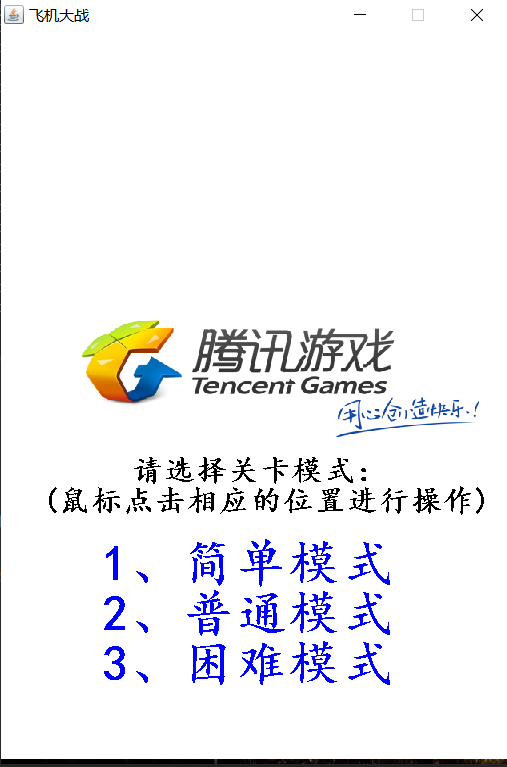﻿ 基于Java语言在窗体上实现飞机大战小游戏的完整步骤_java_脚本之家
java# 基于Java语言在窗体上实现飞机大战小游戏的完整步骤

## 模块需求描述

1. 子弹的创建
2. 子弹的发射
3. 子弹的移动
• 一直向上：中间的子弹
• 左上方向：左边的子弹
• 右上方向：右边的子弹

4.子弹与敌机碰撞

5.子弹的类型升级

• 第一种子弹：伤害值：1
• 第二种子弹：伤害值：2
• 第三种子弹：伤害值：3
• 第四种子弹：伤害值：100

6.子弹的一次发射个数的升级

• 一次发射一个子弹
• 一次发射两个子弹
• 一次发射三个子弹

7.子弹与敌机碰撞

## 总体开发思想

```List<Fire> fires = new ArrayList<Fire>();
```## 功能实现

### 1.登录与结束界面

```// 当游戏结束时，
if (gameover) {
//画游戏登陆时的界面
try {
bg = App.getImg("/img/face.png");
} catch (IOException e) {
printStackTrace();//捕获抛出异常
}

drawImage(bg, 0 ,  0, 512, 728,  null);//画背景图片
//选择关卡模式(鼠标点击相应的位置进行操作)
//画笔为黑色
setColor(Color.black);
//字体大小和类型
setFont(new Font("楷体", Font.BOLD, 30));
//画的内容和位置
drawString("请选择关卡模式：", 130, 450);
drawString("(鼠标点击相应的位置进行操作)", 40, 480);
//画笔为蓝色
setColor(Color.blue);
//字体大小和类型
setFont(new Font("楷体", Font.BOLD, 50));
//画的内容和位置
drawString("1、简单模式", 100, 550);
drawString("2、普通模式" , 100, 600);
drawString("3、困难模式" , 100, 650);
}

```

```//当游戏结束时，读取鼠标的点击位置
if(gameover&&ms==0) {
int x=e.getX();//获取鼠标的横坐标
int y=e.getY();//获取鼠标的纵坐标
if(y>=500&&y<550&&x>=100&&x<400) {
ms=1;//模式 1
}else if(y>=550&&y<600&&x>=100&&x<400) {
ms=2; //模式 2
}else if(y>=600&&y<650&&x>=100&&x<400) {
ms=3; //模式 3
}
// 刷新界面
repaint();
}
```

### 2.播放音乐

```static Player player = null;
static void  play(String str) {
try {
File file = new File(str);//读取文件
FileInputStream fis = new FileInputStream(file);
BufferedInputStream stream = new BufferedInputStream(fis);//读取流的转化
player = new Player(stream);//创建一个播放音乐类对象
player.play();//播放音乐
} catch (FileNotFoundException | JavaLayerException e1) {
printStackTrace();//抛出捕获异常
}
}
```

```/*

*/
//游戏运行音乐
public void music1() {
@Override
public void run() {
while (true) {
System.out.println("播放音乐");
//如果游戏没结束就播放
if (!GamePanel.gameover) {
Music.play("D:\\java文件\\飞机大战.mp3");
}
}
}
} .start();
}
//登陆音乐
public void music2() {
@Override
public void run() {
Music.play("D:\\java文件\\mini.mp3");
}
} .start();
}
```

```//创建音乐类对象
Music music =new Music();
//播放登陆音乐
music.music2();
//播放游戏运行音乐
music.music1();
```

### 3.子弹

```//hx、hy子弹的横纵坐标，dir子弹的移动方向，num子弹的类型
public Fire(int hx,int hy,int dir,int num) throws IOException {
//确定子弹的图片
img=App.getImg("/img/fire"+num+".png");
//确定子弹火力的大小
if(num==1) {
fr=1;
} else if(num==2) {
fr=2;
} else if(num==3) {
fr=3;
} else if(num==4) {
fr=100;
}
w=35;
h=35;
//确定子弹的位置（初始位置在英雄机上）
x=hx;
y=hy;
this.dir=dir;
this.num=num;
}
```

```/*
*创建子弹的方法
*/
int findex = 0;// 记录shoot方法执行的次数
protected void shoot() throws IOException {
findex++;
if (findex == 20) {//减少创建的频率
if (power == 1) {//当火力值为1，即一次发射一颗子弹
// 创建子弹，num子弹的类型
Fire fire3 = new Fire(hero.x + 40, hero.y, 1, num);
// 将子弹加入到弹药库中
//当火力值为2，即一次发射两颗子弹
} else if (power == 2) {
// 创建子弹
Fire fire1 = new Fire(hero.x + 10, hero.y, 1, num);
// 将子弹加入到弹药库中
// 创建子弹
Fire fire2 = new Fire(hero.x + 80, hero.y, 1, num);
// 将子弹加入到弹药库中
//当火力值为3，即一次发射三颗子弹
} else if (power == 3) {
// 创建子弹
Fire fire1 = new Fire(hero.x, hero.y, 0, num);
// 将子弹加入到弹药库中
// 创建子弹
Fire fire2 = new Fire(hero.x + 50, hero.y - 20, 1, num);
// 将子弹加入到弹药库中
// 创建子弹
Fire fire3 = new Fire(hero.x + 100, hero.y, 2, num);
// 将子弹加入到弹药库中
//当火力值为4，即一次发射三颗无敌子弹
} else if (power == 4) {
// 创建子弹
Fire fire1 = new Fire(hero.x+15, hero.y, 0, num);
// 将子弹加入到弹药库中
// 创建子弹
Fire fire2 = new Fire(hero.x, hero.y - 20, 1, num);
// 将子弹加入到弹药库中
// 创建子弹
Fire fire3 = new Fire(hero.x + 70, hero.y, 2, num);
// 将子弹加入到弹药库中
//power置为1，无敌子弹创建完后，变成类型3
power=1;
num=3;
}
findex = 0;// 计数器归零
}
}
-```

```// 在窗体上画子弹
// 遍历集合，画出所有的敌机
for (int i = 0; i < fires.size(); i++) {
// 获取每一个子弹
Fire fire = fires.get(i);
//画出子弹
drawImage(fire.img, fire.x, fire.y, fire.w, fire.h, null);
}
```

```/*
* 子弹移动的方法
*/
public void move() {
if(dir==0) {
//左上
y=y-10;
x=x-2;
}else if(dir==1) {
//直走
y=y-10;
}else if(dir==2) {
//右上
y=y-10;
x=x+2;
}
}
```

```在画板类中：

/*

​    *判断一颗子弹是否击中了敌机

*/
private void bang(Fire fire) {
// 遍历所有的敌机
for (int i = 0; i < eps.size(); i++) {
// 获取每一个敌机
Ep e = eps.get(i);
// 判断该子弹是否击中敌机
if (e.shootBy(fire)) {
// 如果敌机被子弹击中
// 如果不是导弹机(导弹机不会死亡，编号是15)
if (e.type != 15) {
// 1.让敌机的血量减少
hp=e.hp-fire.fr;
// 当敌机的血量减少到零时，死亡
if (e.hp <= 0) {
// 判断敌机是否是道具机（道具机编号为12，打死道具机，子弹会升级）
if (e.type == 12) {
// 增加火力值，即一次发射的子弹数
power++;
// 如果power值大于3,增加英雄机血量，子弹类型升级
if (power > 3) {
hero.hp++;//英雄机增加血量
if (hero.hp > 5) {
hero.hp = 5;
}
//类型1-》类型2
if (num == 1) {
num = 2;
power=1;
//类型2-》类型3
} else if (num == 2) {
num = 3;
power=1;
//类型3-》类型4（创建完子弹类型4后，创建方法后面num置为3，power置为1）
} else if(num==3) {
num=4;
power=4;
}
}
}
// 2.敌机死亡（消失：从敌机大本营中删除）
eps.remove(e);
// 3.增加分数
score =e.type+ score;
//4.消灭的敌机数
Ep.number++;
}
// 4.子弹消失：如果不是类型4（类型4无敌的），就从子弹库中删除
if(num!=4) {
fires.remove(fire);
}
}
}
}
}
```

## 运行测试

### 登陆界面### 发射子弹